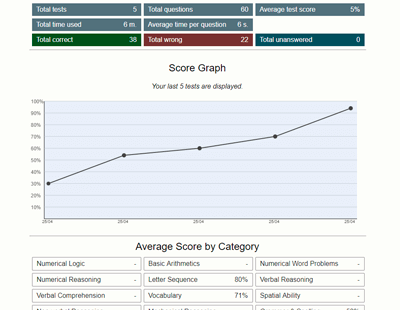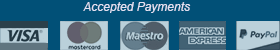﻿ Numerical Word Problems Test - Aptitude-Test.com

# Numerical Word Problems Test

Numerical Word Problems, also simply called math word problems, are mathematical questions presented in a written format. The questions will assess your mathematical ability and your logical reasoning.

What questions can I expect?
The word problems can range from short to long, from simple to complex. Some questions will only require mathematical ability to solve, others will require logical reasoning as well.

Example question:
At a toy factory it takes two machines 50 minutes to create 10 teddy bears. How many teddy bears can one machine create in 30 minutes?

A) 6
B) 5
C) 3
D) 2

Explanation:
First find how many teddy bears one machine can create in 50 minutes:
10 ÷ 2 = 5
Now find how long it takes one machine to create 1 teddy bear:
50 ÷ 5 = 10 minutes per teddy bear At last find how many teddy bears the machine can create in 30 minutes:
30 ÷ 10 = 3 teddy bears

Where are Numerical Word Problems tests used?
Numerical Word Problems can be found in pre-employment tests for jobs requiring math skills in day-to-day functions, including cashiers, analysts, bank tellers, engineers, and more.## Practice Numerical Word Problems Test

Try a free Numerical Word Problems Test.
This free practice test contains 6 test questions and has a time limit of 9 minutes.

##### Would you like to improve your test score? Practice smart with a Test Prep Account.

Practice on 120 Numerical Word Problems and a total of 530 numerical aptitude test questions with detailed description and score statistics.

The many features of a Test Prep Account:

• Preparation software developed under a didactic and methodical perspective
• High-quality practice test questions
• Clearly explained solutions
• Accessible on all devices
• Detailed score data and progression charts
• Reference scores to compare your performance against others
• Training Assistant
• Practice mode (feedback after every question and no time limit)